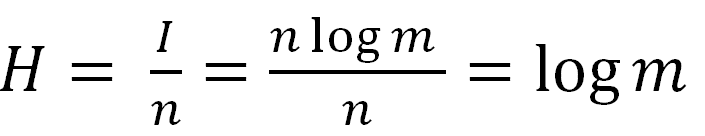Search
• Alibek Jakupov

# Information Theory: Step 1 Probability

Updated: May 17, 2020## Introduction

This article is the first part of a series of articles devoted to the study of the Information Theory, step by step. In this article are going to introduce the topic and provide a simple code for the first part.

The aim is to get acquainted with the basics of data transmission by investigating this field of information theory, and to broaden the general understanding of the principles and laws of data encoding.

Information theory is a branch of applied mathematics, electrical engineering, and computer science involving the quantification of information. Information theory was developed by Claude E. Shannon to find fundamental limits on signal processing operations such as compressing data and on reliably storing and communicating data. Since its inception it has broadened to find applications in many other areas, including statistical inference, natural language processing, cryptography, neurobiology, the evolution and function of molecular codes, model selection in ecology, thermal physics, quantum computing, linguistics, plagiarism detection, pattern recognition, anomaly detection and other forms of data analysis.

Key points abount information

• Measurement

• Redundancy

• Entropy

• Transfer

• Coding

• Decoding

• Modulation

• Demodulation

Method of information encoding depends on the objective:

• reducing the length of a record – optimal coding;

• protection against noise during the transmission of information - error-correcting coding; hiding information - data encryption.

Dr. Daniel Polani, Professor of Artificial Intelligence, University of Hertfordshire

To put it saucily: information theory is something like the logarithm of probability theory. In early modern times the logarithm simplified multiplication into addition which was more accessible to calculation. Today, information theory transforms many quantities of probability theory into quantities which allow simpler bookkeeping. More seriously, information theory is one of the most universal concepts with applications in computer science, mathematics, physics, biology, chemistry and other fields. It allows a lucid and transparent analysis of many systems and provides a framework to study and compare seemingly different systems using the same language and notions.

## Probability theory

An alphabet of message source consists of m characters, each of which can be a message element. The N number of possible messages of n length equals to the number of permutations with unlimited repetitions:Logarithm of states number is taken as a measure of the uncertainty of the source state selection with equiprobable states.Hartley’s formula (1928)K – number of equiprobable events;

I – the number of bits in the message that any of the K events happened.

## Entropy

Entropy is a measure of uncertainty.

Information is a measure of a reduction in that uncertainty.

The information of the message:

I = H0-H1

H0 – the entropy before the message

H1 – the entropy after the message

Thus, the amount of information that falls on one element of the message (sign, letter) is called entropy:## Shannon’s formula

If

1. I – amount of information,

2. K – number of possible events,

3. pi – probabilities of individual events,

the amount of information for events with different probabilities can be determined by the formula:where I is from 1to K.

N.B. Hartley’s formula can be seen as a particular case of Shannon’s formula. •If events are equiprobable we get a maximum of information.

## Practice:

As we have seen before, the first step is to find probabilities. The probabilities are necessary to find out in order to calculate the letter weights and construct a binary tree (to implement the Hamming algorithm - see in next articles).

Here is the sample code needed to find probabilities:

```#Init class to store the collection of letters
import math

class Letter:
def __init__(self, letter, quantity, msgSize) :
self.letter = letter
self.quantity = float(quantity)
self.msgSize = float(msgSize)
def getLetter(self):
return self.letter
def getQuantity(self):
return self.quantity
def getMsgSize(self):
return self.msgSize
def getProbabilty(self):
return float(self.quantity/self.msgSize)

a =[]
temp = []
x = raw_input("Please, enter your sequence:")
length = len(x)
for i in x:
if temp.count(i) == 0:
tempObj = Letter(i, x.count(i), length)
a.append(tempObj)
temp.append(i)
print temp
for item in a:
print item.getLetter()
print item.getProbabilty()```

Code is also available here

## Books:

Arndt, C. Information Measures: Information and its Description in Science and Engineering / C. Arndt.- 2nd.- Germany: Springer, 2004.- 547p.